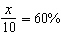Name:    Chapter 3 Test

Multiple Choice
Identify the choice that best completes the statement or answers the question.

Use the table to find the rate.

1.

 Minutes 0 1 2 3 Gallons 0 1.4 2.8 4.2
 a. 2.8 minutes per gallon c. 1.4 gallons per minute b. 1.4 minutes per gallon d. 2.8 gallons per minute

2.

 Batches 5 10 15 20 Servings 12.5 25 37.5 50
 a. 12.5 batches per serving c. 12.5 servings per batch b. 2.5 batches per serving d. 2.5 servings per batch

3.

 Gallons 4 9 14 19 Miles 94.4 212.4 330.4 448.4
 a. 94.4 miles per gallon c. 23.6 gallons per mile b. 94.4 gallons per mile d. 23.6 miles per gallon

4.

At 7 P.M., a burning candle is 16 inches tall. At 9:30 P.M., the candle is only 4.75 inches tall. At what rate is the height of the candle decreasing?
 a. 1.9 in. per h c. 6.4 in. per h b. 11.25 in. per h d. 4.5 in. per h

Find the slope of the line.

5.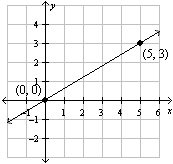a. 3 c.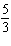b. 5 d.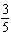6.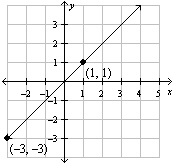a.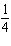c. 4 b.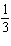d. 1

Use the table to write a proportion.

7.

 Race 1 Race 2 Meters 40 120 Seconds 7 s
 a.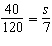c.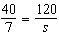b.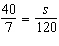d.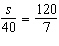Solve the proportion using multiplication.

8.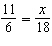a. x = 33 c. x = 36 b. x = 37 d. x = 32

9.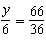a. y = 12 c. y = 8 b. y = 11 d. y = 9

10.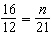a. n = 28 c. n = 31 b. n = 15.8 d. n = 25

11.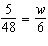a. w =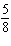c. w =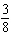b. w =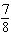d. w =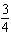12.

You are planning a party. Your friend tells you how much she paid for five party grab bags. How much will it cost you to buy nine of the grab bags for your party?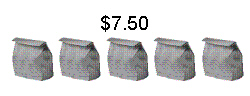a. \$12.00 c. \$21.00 b. \$67.50 d. \$13.50

Solve.

13.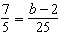a. b = 185 c. b = 33 b. b = 35 d. b = 37

14.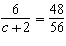a. c = 5 c. c = 6 b. c = 9 d. c = 7

15.

The species Myotis lucifugus is commonly known as the “little brown bat.” An average little brown bat weighsounce. As many as 300,000 little brown bats may share the same roosting location.  How many kilograms would 300,000 little brown bats weigh?
 a. 100,000 kg c. 2812.5 kg b. 13,888.9 kg d. 8437.5 kg

The variables x and y vary inversely.  Write an equation relating x and y.

16.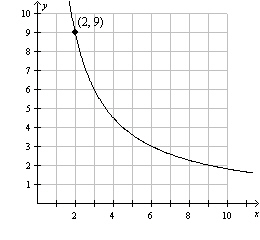a.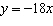c.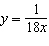b.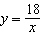d.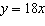Numeric Response

Solve the proportion using the Cross Products Property.

1.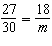2.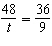Tell whether the ratios form a proportion.

1.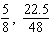2.

Explain how to rewrite the equation as a proportion. Then write the proportion.